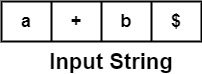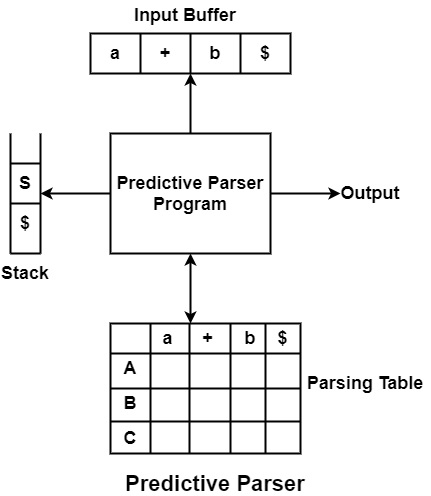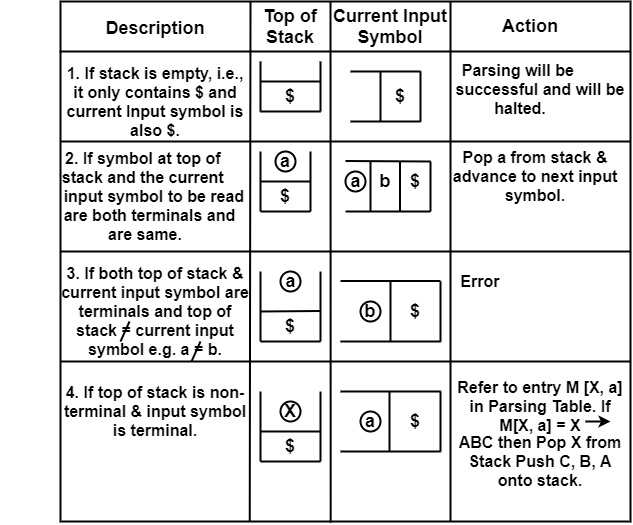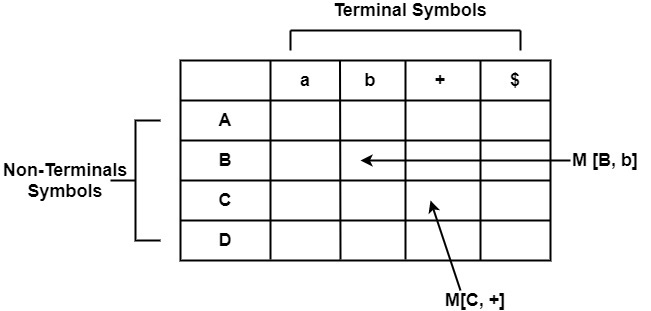# What is a Predictive Parser?

Predictive Parser is also another method that implements the technique of Top- Down parsing without Backtracking. A predictive parser is an effective technique of executing recursive-descent parsing by managing the stack of activation records, particularly.

Predictive Parsers has the following components −

• Input Buffer − The input buffer includes the string to be parsed followed by an end marker $to denote the end of the string.Here a, +, b are terminal symbols. • Stack − It contains a combination of grammar symbols with$ on the bottom of the stack. At the start of Parsing, the stack contains the start symbol of Grammar followed by $.• Parsing Table − It is a two-dimensional array or Matrix M [A, a] where A is nonterminal and 'a' is a terminal symbol. All the terminals are written column-wise, and all the Non-terminals are written rowwise. • Parsing Program − The parsing program performs some action by comparing the symbol on top of the stack and the current input symbol to be read on the input buffer. • Actions − Parsing program takes various actions depending upon the symbol on the top of the stack and the current input symbol. Various Actions taken are given below −Algorithm to construct Predictive Parsing Table Input − Context-Free Grammar G Output − Predictive Parsing Table M Method − For the production A → α of Grammar G. • For each terminal, a in FIRST (𝛼) add A → α to M [A, a]. • If ε is in FIRST (α), and b is in FOLLOW (A), then add A → α to M[A, b]. • If ε is in FIRST (α), and$ is in FOLLOW (A), then add A → α to M[A, \$].
• All remaining entries in Table M are errors.Following are the steps to perform Predictive Parsing

• Elimination of Left Recursion
• Left Factoring
• Computation of FIRST & FOLLOW
• Construction of Predictive Parsing Table
• Parse the Input String